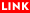## Professor　KASUE Atsushi### Faculty, Affiliation

Faculty of Mathematics and Physics, Institute of Science and Engineering

### College and School Educational Field

Division of Mathematical and Physical Science, Graduate School of Natural Science and Technology
Division of Mathematical and Physical Sciences, Graduate School of Natural Science and Technology
School of Mathematics and Physics, College of Science and Engineering

### Laboratory

Department of Mathematics　TEL:076-264-5643　FAX:076-264-5738

Osaka University　Master　1980
Osaka University　1978

1954/07

### Specialities

Laplace operator Spectral convergence、differential geometry

### Speciality Keywords

Riemannian manifold, network, energy form, Laplace operator, potential theory

### Research Themes

#### Compactifications of Riemannian manifolds and embeddings of graphs

We study p-harmonic functions of finite p-Dirichlet sum
on networks in Hadamard manifolds in relation to their
geometric compactifications and embeddings of finite
graphs into the maifolds.

#### Dirichlet finite maps and asymptotic geometry of infinite graphs

We investigate the spaces of functions of finite Dirichlet sums in indinite graphs or
infinite networks in relation to geometric structure of graphs or networks,via quasimono-morphisms or Dirichlet finite maps. Our study is focuced on behavior of such maps near Royden boundary, Kuramochi boundary, Floyd boundary, and Gromov hyperbolic boun-
dary of graphs or networks. It is important to treat with general exponents other than 2
in Dirichlet finite functions or maps.

#### Convergence theory of metric measure spaces

I study sets of finite networks (weighted graphs). By using effective resistance (combined resistance), Hausdorff convergence in the sense of Gromov and variation convergence based on energy form can be defined. A variety of things appear in the diagrams (spaces) that emerge in the limit of the sets based in these phases, including so-called fractal sets and infinite networks. The present research analyzes the Markov-type family that appears in the limit and Kuramochi compactization associated with each, primarily focusing on infinite networks (reversible Markov chains).

#### Random walks and Dirichlet finite maps

I study the relationship between random walk on an infinite network, and Dirichlet energy finite function. More generally, I research Dirichlet energy finite mapping onto complete metric space. Dirichlet energy finite mapping, when followed along random walk, approaches a finite definite value. I investigate the relationship between this fact and the space called Kuramochi compactization of infinite network.

### Books

• 　Riemmannian manifolds and their limits　Mathematical Society of Japan　2004/08

### Papers

• 　Convergence of Dirichlet forms induced on boundarieis of transient networks　Atsushi Kasue　Potential Analysis　47 　2　189-233　2017/02/01
• 　Random walks and Kuramochi boundaries of infinite networks　OSAKA JOURNAL OF MATHEMATICS　50　1　31-51　2013/03
• 　Functions with finite Dirichlet sum of order p and quasi-monomorphisms of infinite graphs 　Tae Hattori　NAGOYA MATHEMATICAL JOURNAL　207　95-138　2012/08
• 　Covergence of metric graphs and energy forms　26　2　367-448　2010/04
• 　Convergence of Riemannian manifolds and Laplace operators, II　POTENTIAL ANALYSIS　24　137-194　2006/01
• 　Variational Convergence of Finite Networks　12　1　57-70　2006
• 　A Thmson's principle and a Rayleigh's monotonicity law for nonlinear networks　Atsushi Kasue　Potential Analysis　2016/06/06
• 　Dirichlet finite harmonic functions and points at infinity of graphs and manifolds,　Tae Hattori　PROCEEDINGS OF THE JAPAN ACADEMY SERIES A-MATHEMATICAL SCIENCES　83　129-134　2007
• 　EXPANSION CONSTANTS AND HYPERBOLIC EMBEDDINGS OF FINITE GRAPHS　Hattori, Tae; Kasue, Atsushi　Mathematika　2014/11/19### Grant-in-Aid for Scientific Research

○「倉持境界の幾何解析の展開」(2016-2019)
○「測度距離空間の収束理論とその展開」(2007-)
○「測度距離空間の収束とエネルギー形式」(2003-)
○「リーマン多様体の収束とラプラス作用素」(2001-)
○「リーマン多様体のコンパクト化とグラフの埋め込み」(2012-2015)

### Classes (Bachelors)

○Mathematical Thinking(2017)
○Geometry 2A(2017)
○Geometry 2B(2017)
○Analysis 2C(2017)
○Mathematical Thinking(2017)
○Differential and Integral Calculus 1(2016)
○Mathematical Thinking(2016)
○Geometry 2A(2016)
○Geometry 2B(2016)
○Introduction to Current Mathematics(2016)

### Classes (Graduate Schools)

○Geometric Analysis(2017)
○Exercise A(2017)
○Seminar A(2017)
○Research Work A(2017)
○Mathematics Education b(2016)
○Mathematics Education a(2016)
○Research Work A(2016)
○Exercise A(2016)
○Seminar A(2016)
○Geometric Analysis(2016)

### Lecture themes

○Lines, circles and elementary geometry

To Page Top